# Fraction calculator

The calculator performs basic and advanced operations with fractions, expressions with fractions combined with integers, decimals, and mixed numbers. It also shows detailed step-by-step information about the fraction calculation procedure. Solve problems with two, three, or more fractions and numbers in one expression.

## Result:

### 45/8 + 23/4 = 59/8 = 7 3/8 = 7.375

Spelled result in words is fifty-nine eighths (or seven and three eighths).

### How do you solve fractions step by step?

1. Conversion a mixed number 4 5/8 to a improper fraction: 4 5/8 = 4 5/8 = 4 · 8 + 5/8 = 32 + 5/8 = 37/8

To find a new numerator:
a) Multiply the whole number 4 by the denominator 8. Whole number 4 equally 4 * 8/8 = 32/8
b) Add the answer from previous step 32 to the numerator 5. New numerator is 32 + 5 = 37
c) Write a previous answer (new numerator 37) over the denominator 8.

Four and five eighths is thirty-seven eighths
2. Conversion a mixed number 2 3/4 to a improper fraction: 2 3/4 = 2 3/4 = 2 · 4 + 3/4 = 8 + 3/4 = 11/4

To find a new numerator:
a) Multiply the whole number 2 by the denominator 4. Whole number 2 equally 2 * 4/4 = 8/4
b) Add the answer from previous step 8 to the numerator 3. New numerator is 8 + 3 = 11
c) Write a previous answer (new numerator 11) over the denominator 4.

Two and three quarters is eleven quarters
3. Add: 37/8 + 11/4 = 37/8 + 11 · 2/4 · 2 = 37/8 + 22/8 = 37 + 22/8 = 59/8
For adding, subtracting, and comparing fractions, it is suitable to adjust both fractions to a common (equal, identical) denominator. The common denominator you can calculate as the least common multiple of both denominators - LCM(8, 4) = 8. In practice, it is enough to find the common denominator (not necessarily the lowest) by multiplying the denominators: 8 × 4 = 32. In the following intermediate step, the fraction result cannot be further simplified by canceling.
In other words - thirty-seven eighths plus eleven quarters = fifty-nine eighths.

#### Rules for expressions with fractions:

Fractions - use the slash “/” between the numerator and denominator, i.e., for five-hundredths, enter 5/100. If you are using mixed numbers, be sure to leave a single space between the whole and fraction part.
The slash separates the numerator (number above a fraction line) and denominator (number below).

Mixed numerals (mixed fractions or mixed numbers) write as non-zero integer separated by one space and fraction i.e., 1 2/3 (having the same sign). An example of a negative mixed fraction: -5 1/2.
Because slash is both signs for fraction line and division, we recommended use colon (:) as the operator of division fractions i.e., 1/2 : 3.

Decimals (decimal numbers) enter with a decimal point . and they are automatically converted to fractions - i.e. 1.45.

The colon : and slash / is the symbol of division. Can be used to divide mixed numbers 1 2/3 : 4 3/8 or can be used for write complex fractions i.e. 1/2 : 1/3.
An asterisk * or × is the symbol for multiplication.
Plus + is addition, minus sign - is subtraction and ()[] is mathematical parentheses.
The exponentiation/power symbol is ^ - for example: (7/8-4/5)^2 = (7/8-4/5)2

#### Examples:

subtracting fractions: 2/3 - 1/2
multiplying fractions: 7/8 * 3/9
dividing Fractions: 1/2 : 3/4
exponentiation of fraction: 3/5^3
fractional exponents: 16 ^ 1/2
adding fractions and mixed numbers: 8/5 + 6 2/7
dividing integer and fraction: 5 ÷ 1/2
complex fractions: 5/8 : 2 2/3
decimal to fraction: 0.625
Fraction to Decimal: 1/4
Fraction to Percent: 1/8 %
comparing fractions: 1/4 2/3
multiplying a fraction by a whole number: 6 * 3/4
square root of a fraction: sqrt(1/16)
reducing or simplifying the fraction (simplification) - dividing the numerator and denominator of a fraction by the same non-zero number - equivalent fraction: 4/22
expression with brackets: 1/3 * (1/2 - 3 3/8)
compound fraction: 3/4 of 5/7
fractions multiple: 2/3 of 3/5
divide to find the quotient: 3/5 ÷ 2/3

The calculator follows well-known rules for order of operations. The most common mnemonics for remembering this order of operations are:
PEMDAS - Parentheses, Exponents, Multiplication, Division, Addition, Subtraction.
BEDMAS - Brackets, Exponents, Division, Multiplication, Addition, Subtraction
BODMAS - Brackets, Of or Order, Division, Multiplication, Addition, Subtraction.
GEMDAS - Grouping Symbols - brackets (){}, Exponents, Multiplication, Division, Addition, Subtraction.
Be careful, always do multiplication and division before addition and subtraction. Some operators (+ and -) and (* and /) has the same priority and then must evaluate from left to right.

## Fractions in word problems:Max is working out 2/3+7/9. He says the answer is 9/12. What mistake have Max made?7 is added to the sum of 4/5 and 6/7
• Samuel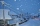Samuel has 1/3 of a bag of rice and Isabella has a 1/2 bag of rice. What fraction of are bag of rice do they have altogether?
• Patel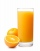Patel squeezed oranges so that his family could have fresh-squeezed juice for breakfast. He squeezed 4/17 cups from the first orange, 3/10 cups from the second orange, StartFraction 9 over 20 E
• Berry SmoothieRory has 5/8 cup of milk. How much milk does she have left after she doubles the recipe of the smoothie? Berry Smoothie: 2 cups strawberries 1 cup blueberries 1/4 cup milk 1 tbsp (tablespoon) sugar 1/2 tsp (teaspoon) lemon juice 1/8 tsp (teaspoon) vanillaPeter bought 1/2 a pound of chocolate at rocky mountain chocolate factory. Later he went to the sweet shoppie and he bought 6/9 of a pound more chocolate. How much chocolate did he buy that day?
• How many 3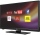How many hours the Andersons watched TV in all Wednesday 3/1 hr Thursdays 2/3 hr Friday 4/5 hr Saturday 3/4 hr
• Cups of flour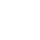Jade was baking cupcakes for her class. She has 4 5/4 cups of flour.  For one batch, she used 1 2/3 cups of flour.  On another batch, she used 7/9 of a cup of flour.  How much flour does Jade have left after making the two batches of cupcakes?
• Simplify 9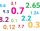Simplify and express the result as a rational number in its simplest form 1/2+ 1/5+ 6.25+0.25
• A dumpA dump truck bought 1/3 of a ton of rock on the first trip, 1/2 of a ton on the second trip, and 4/5 of a ton on the third trip. What was the total weight of the rock?The weight of the empty fruit basket is ¾ kg. We added ½ kg apples and 5/4 kg guavas. What will be the total weight of the fruit basket?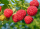Carrie picked 2/5 of the raspberries from the garden, and Robin picked some too.  When they were finished, 1/3 of the raspberries still needed to be picked.  What fraction of the raspberries did Robin pick? Use pictures, numbers or words and write your fi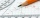Calculate the sum of 1/5 of a right angle and 3/4 of a right angle and 3/4 of a straight angle